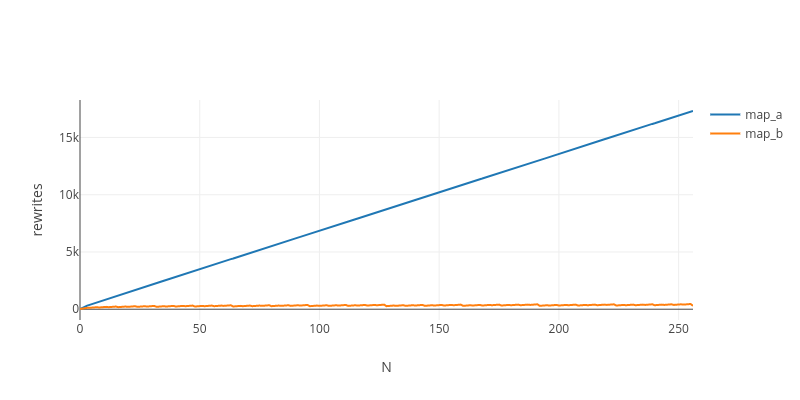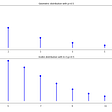# Solving the mystery behind Abstract Algorithm’s magical optimizations

## 1. Optimizable functions are those that fuse when self-composed

`True  = λt. λf. tFalse = λt. λf. fnot_a = λb. (b False True)not_b = λb. λt. λf. (b f t)`
• norm(`not_a.not_a`): `λb. (b (λt.λf.f) (λt.λf.t) (λt.λf.f) (λt.λf.t))`
• norm(`not_b.not_b`): `λb. λt. λf. (b t f)`
`Nil   =         λc. λn. nCons  = λh. λt. λc. λn. c h (t c n)map_a = λf. λl.         l (λh. λt. Cons (f h) t) Nilmap_b = λf. λl. λc. λn. l (λh. λt. c    (f h) t) nlist  = Cons True (Cons False (Cons True (Cons False Nil)))`Complexity (total rewrites) of applying “map” N times to a list of 4 elements

## 2. Exponentiation by squaring = composition by sharing

`# Computing 13^8 with repeated multiplication13^2 = 13 * 13 = 16913^3 = 13 * 169 = 219713^4 = 13 * 2197 = 2856113^5 = 13 * 28561 = 37129313^6 = 13 * 371293 = 482680913^7 = 13 * 4826809 = 6274851713^8 = 13 * 62748517 = 815730721# Computing 13^8 with exponentiation by squaring13^2 = 13 * 13 = 16913^4 = 169 * 169 = 2856113^8 = 28561 * 28561 = 815730721`
`# Computing not^8(t) with repeated applicationnot^2(t) = (λb.λf.λt. b f t) . (λb.λf.λt. b f t) = (λb.λf.λt. b t f)not^3(t) = (λb.λf.λt. b f t) . (λb.λf.λt. b t f) = (λb.λf.λt. b f t)not^4(t) = (λb.λf.λt. b f t) . (λb.λf.λt. b f t) = (λb.λf.λt. b t f)not^5(t) = (λb.λf.λt. b f t) . (λb.λf.λt. b t f) = (λb.λf.λt. b f t)not^6(t) = (λb.λf.λt. b f t) . (λb.λf.λt. b f t) = (λb.λf.λt. b t f)not^7(t) = (λb.λf.λt. b f t) . (λb.λf.λt. b t f) = (λb.λf.λt. b f t)not^8(t) = (λb.λf.λt. b f t) . (λb.λf.λt. b f t) = (λb.λf.λt. b t f)# Computing not^8(t) with composition by sharingnot^2(t) = (λb.λf.λt. b f t) . (λb.λf.λt. b f t) = (λb.λf.λt. b t f)not^4(t) = (λb.λf.λt. b t f) . (λb.λf.λt. b t f) = (λb.λf.λt. b t f)not^8(t) = (λb.λf.λt. b t f) . (λb.λf.λt. b t f) = (λb.λf.λt. b t f)`
`# Computing not^8(x) with repeated applicationnot^2(x) = not . not = id not^3(x) = not . id  = notnot^4(x) = not . not = id not^5(x) = not . id  = notnot^6(x) = not . not = id not^7(x) = not . id  = notnot^8(x) = not . not = id# Computing not^8(x) with composition by sharingnot^2(x) = not . not = id not^4(x) = id  . id  = id not^8(x) = id  . id  = id`

# But what about the negative complexity stuff?

`-- Normal form of `inc`:λa. λb. λc. λd. (((a c) λe. (b λf. λg. λh. (((e g) λi. (f λj.λk. λl. (((i k) λm. (j m)) l))) h))) d)-- Normal form of `inc . inc`:λa. λb. λc. λd. (((a λe. (b λf. λg. λh. (((e g) λi. (f λj. λk. λl. (((i k) λm. (j m)) l))) h))) λe. (c λf. λg. λh. (((e g)λi. (f λj. λk. λl. (((i k) λm. (j m)) l))) h))) d)-- Normal form of `inc . inc . inc`:λa. λb. λc. λd. (((a λe. (c λf. λg. λh. (((e g) λi. (f λj. λk. λl. (((i k) λm. (j m)) l))) h))) λe. (b λf. λg. λh. (((e λi. (f λj. λk. λl. (((i k) λm. (j m)) l))) λi. (g λj. λk. λl. (((i k) λm. (j m)) l))) h))) d)--------------------------------------------------------------- Normal form of `copy . inc`:λa. (((a λb. λc. λd. λe. (d (((b λf. λg. λh. λi. (g (((f λj. λk. λl. λm. (k j)) λj. λk. λl. λm. (l j)) λj. λk. λl. l))) λf. λg. λh. λi. (h (((f λj. λk. λl. λm. (k j)) λj. λk. λl. λm. (l j)) λj. λk. λl. l))) λf. λg. λh. h))) λb. λc. λd. λe. (c (((b λf. λg. λh. λi. (h (((f λj. λk. λl. λm. (k j)) λj. λk. λl. λm. (l j)) λj. λk. λl. l))) λf. λg. λh. λi. (g (((f λj. λk.λl. λm. (l j)) λj. λk. λl. λm. (k j)) λj. λk. λl. l))) λf. λg. λh. h))) λb. λc. λd. d)-- Normal form of `copy . inc . inc`:λa. (((a λb. λc. λd. λe. (c (((b λf. λg. λh. λi. (h (((f λj. λk. λl. λm. (k j)) λj. λk. λl. λm. (l j)) λj. λk. λl. l))) λf. λg. λh. λi. (g (((f λj. λk. λl. λm. (l j)) λj. λk. λl. λm. (k j)) λj. λk. λl. l))) λf. λg. λh. h))) λb. λc. λd. λe. (d (((b λf. λg. λh. λi. (h (((f λj. λk. λl. λm. (k j)) λj. λk. λl. λm. (l j)) λj. λk. λl. l))) λf. λg. λh. λi. (g (((f λj. λk.λl. λm. (l j)) λj. λk. λl. λm. (k j)) λj. λk. λl. l))) λf. λg. λh. h))) λb. λc. λd. d)-- Normal form of `copy . inc . inc . inc`:λa. (((a λb. λc. λd. λe. (d (((b λf. λg. λh. λi. (h (((f λj. λk. λl. λm. (k j)) λj. λk. λl. λm. (l j)) λj. λk. λl. l))) λf. λg. λh. λi. (g (((f λj. λk. λl. λm. (l j)) λj. λk. λl. λm. (k j)) λj. λk. λl. l))) λf. λg. λh. h))) λb. λc. λd. λe. (c (((b λf. λg. λh. λi. (g (((f λj. λk. λl. λm. (l j)) λj. λk. λl. λm. (k j)) λj. λk. λl. l))) λf. λg. λh. λi. (h (((f λj. λk.λl. λm. (l j)) λj. λk. λl. λm. (k j)) λj. λk. λl. l))) λf. λg. λh. h))) λb. λc. λd. d)-- Note: using a fixed depth of 3 for better visualization`

## tl;dr

`λf1. λx.   let f2  = f1 . f1 in   let f4  = f2 . f2 in  let f8  = f4 . f4 in  let f16 = f8 . f8 in  f16 (f4 (f1 x))`

--

--

--

## More from Victor Maia

I’m something that possibly exists

Love podcasts or audiobooks? Learn on the go with our new app.

## NBA playoff win chances via Bayesian inference## HHL: Solving Linear Systems of Equations with Quantum Computing## When Bayes, Ockham, and Shannon come together to define machine learning## Exact Diagonalization Put Briefly.## A Shot of Scotch #4: Göring Gambit | Chess Openings Explained## Scientific Computation using Matlab: Week 1## Understanding Geometric and Inverse Binomial distribution## Monty Hall Problem solved with Python## Victor Maia

I’m something that possibly exists

## 3 Fundamentals of Enums in Rust## Data Structures in Typescript and Rust with Borsh## Part #2: How to read WASM